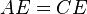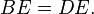# Parallelogram facts for kids

Kids Encyclopedia Facts

A parallelogram is a polygon with four sides (a quadrilateral). It has two pairs of parallel sides (sides which never meet) and four edges. The opposite sides of a parallelogram have the same length (they are equally long). The word "parallelogram" comes from the Greek word "parallelogrammon" (bounded by parallel lines). Rectangles, rhombuses, and squares are all parallelograms.

As shown in the picture on the right, because triangles ABE and CDE are congruent (have the same shape and size),$AE = CE$$BE = DE.$

In all Parallelogram's opposite angles are equal to each other. Angles which are not opposite in the Parallelogram will add up to 180 degrees.

## Characterizations

A simple (non self-intersecting) quadrilateral is a parallelogram if and only if any one of the following statements is true:

• Two pairs of opposite sides are equal in length
• Two pairs of opposite angles are equal in measure
• The diagonals bisect each other
• One pair of opposite sides are parallel and equal in length